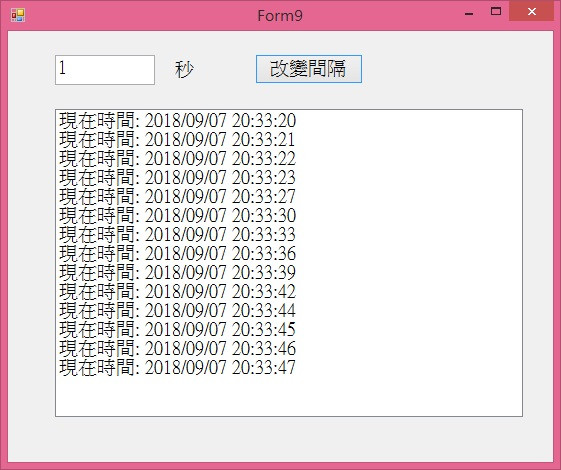#0

## C# 請問 一個 timer 小語法

`````` InitializeComponent();
int time = Convert.ToInt32(textBox1.ToString());
timer1.Interval = 1000 * time;
timer1.Enabled = true;
timer1.Start();
``````

`````` timer1.Interval = 1000;
``````

``````int time = Convert.ToInt32(textBox1.ToString());
timer1.Interval = 1000 * time;
``````

1.給textbox.text預設值
2.判斷textbox.text有沒有值,如果沒有值,給預設值
ted8224 iT邦新手 5 級 ‧ 2018-09-07 13:42:10 檢舉
textBox1.Text ="0";

textBox1.text

string stext=textBox1.text;
if (IsNullOrEmpty(stext))
stext="0";

1.不可以寫在初始化

2.是textBox1.Text.ToString()不是textBox1.ToString()

var a = textBox1.ToString();
var b = Convert.ToInt32(a);

### 4 個回答

0

ted8224 iT邦新手 5 級 ‧ 2018-09-07 13:58:33 檢舉

``````Convert.ToInt32
``````
0

NumericUpDown

TextBox ，您還要去確認是否輸入的是數字，還是空白+ 數字

0``````private void button1_Click(object sender, EventArgs e)
{
int number = 0;
if(Int32.TryParse(textBox1.Text, out number))
{
timer1.Interval = number * 1000;
}
else
{
MessageBox.Show("請輸入數字");
}
}
``````

Convert.ToInt32如果遇到無法轉換的，就會拋出例外。如果沒有用Try Catch包起來，會導致程式Crash

ted8224 iT邦新手 5 級 ‧ 2018-09-08 22:27:19 檢舉

0

int time = Convert.ToInt32(textBox1.ToString());

ted8224 iT邦新手 5 級 ‧ 2018-09-08 22:26:39 檢舉
``````int time = Convert.ToInt32(textBox1.Text.ToString());
``````